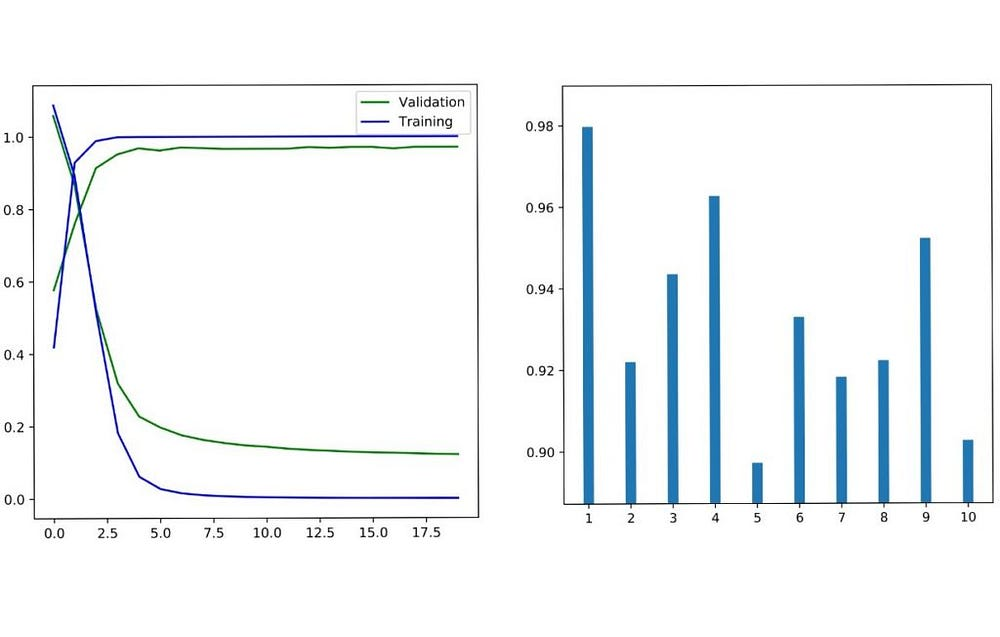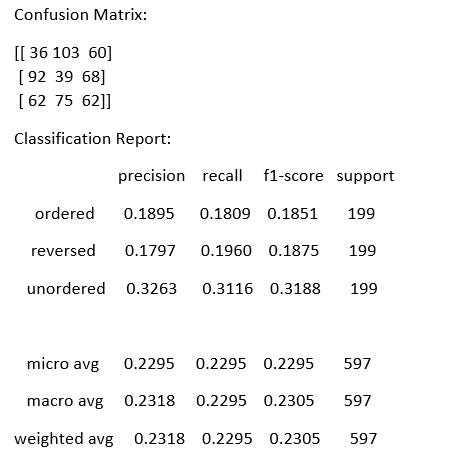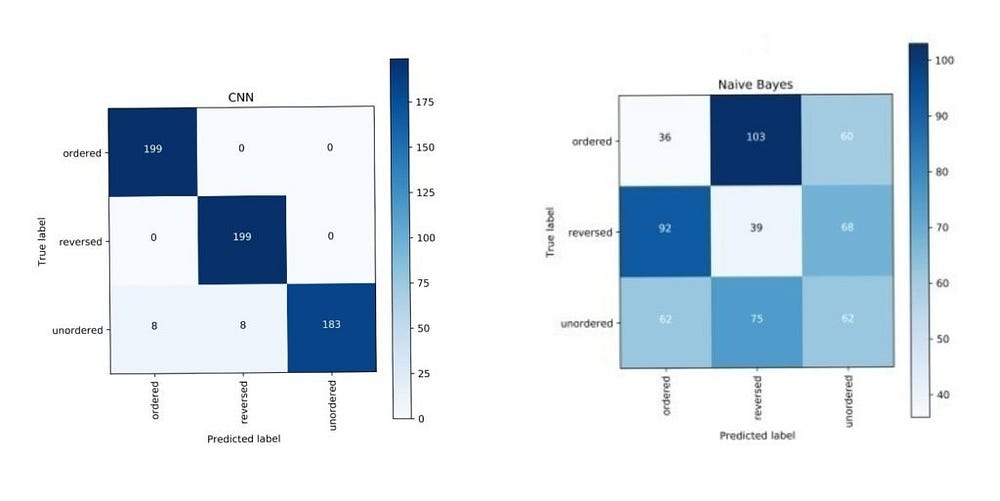# Sequence Based Text Classification with Convolution Nets

Source: Deep Learning on Medium

Apparently Feynman once said about the universe, “it’s not complicated, it’s just a lot of it“. A machine might be thinking the same, while dealing with a plethora of words and trying to make sense of relations between them. We use and interpret words or sentences in a context. A lot of context arises from a sequence. Unfortunately, infinitely many sequences of words are possible and most of them are gibberish and yield no context. You might have seen with some approaches like bag of words, we usually side-step this complexity by ignoring the specific sequences of words altogether and instead treating documents as bags of words as per the VSM approach. But looks like there are some new generation models in town (or at least feels like new, even though they have existed for decades) that could account for sequences of words without breaking the bank, and we’re going to see one of those in action today.

The problem with approaches like bag of words, document vector approach is that these techniques don’t emphasize on the sequence of the words and their respective occurrences or relations. Two sentences could have same representation but totally different meaning. Let’s consider one sentence: ‘Thank you for helping me’, now by altering a few words it could be written as, ‘You thank me for helping’. This rearrangement of words though makes a completely new sentence but it’ll be observed as the same when viewed from bag of words approach.

This is where we need such a model which extracts the relation or patterns from a group of words, not from an individual word. In such a scenario, Convolutional Neural Networks (CNN) can produce some really good results. CNNs are known for extracting the patterns from the underlying sentences by focusing on a sequence of words at a time.

### What is CNN?

Before moving on to the original classification task let’s discuss in brief about Convolution Nets. CNN i.e. a ‘Convolutional Neural Network’ is a class of deep, feed-forward artificial neural networks, most commonly used for analyzing images. These networks use a variation of multi-layer perceptrons designed to minimal pre-processing.

A typical CNN model consists of an input and an output layer, as well as multiple hidden layers. The hidden layers of a CNN consists of convolutional layers, pooling layers, fully connected layers and normalization layers (optional).

Convolution layers receives the input and it uses a convolution filter to convolve over the underlying data points to extract meaningful patterns out of it. For example in case of a 2D-image, this will look something like this:

This extracted set of features are then passed to MaxPooling layer, which filters the dominating terms from the extracted features. The illustration for that would look like this:

These filtered terms are then passed onto the final layers before classification, which are flatten & fully connected layers. And the output of fully connected layers are what we see as the final classification results.

So, with this post we are trying to classify custom text sequences using CNN and Naive Bayes with tf-idf for comparison. CNN here is implemented with the help of Keras and tensorflow as its back-end. For Naive bayes we are using sklearn or scikit-learn.

### Implementation:

#### Generating Custom text data:

The reason for choosing such a custom corpus is to demonstrate the difference between bag of words approach with Naive Bayes and sequence based approach with convolution nets. You can find all code snippets, including the data generating ones by visiting this GitHub repository link.

Now we’ll see how encoding and CNN part are implemented to obtain the final results. The main imports for this task are as follows:

import numpy as np
import os
import json
from sklearn.metrics import classification_report, confusion_matrix
from sklearn.model_selection import StratifiedShuffleSplit
import random as rn
import keras
import tensorflow as tf
os.environ[‘TF_CPP_MIN_LOG_LEVEL’]=’2′

In the code snippet below, we fix some random seeds for tensorflow and numpy so that we can get some reproducible results.

#All this for reproducibility
np.random.seed(1)
rn.seed(1)
tf.set_random_seed(1)
sess = tf.Session(graph=tf.get_default_graph(), config=session_conf)
keras.backend.set_session(sess)

#### Encoding:

Use the Keras text processor on all the sentences/sequences so it can generate a word index and encode each sequence (Lines 2–4 below) accordingly. Note that we do not need padding as the length of all our sequences is exactly 15.

# Encode the documents
kTokenizer = keras.preprocessing.text.Tokenizer()
kTokenizer.fit_on_texts(X)
Xencoded = np.array([np.array(xi) for xi in kTokenizer.texts_to_sequences(X)])
labels = np.array(labels)

#### CNN Implementation:

CNN implementation will consist of the embedding layer, convolution layer, pooling layer (we’ll use MaxPooling here), one flatten layer and the final dense layer or the output layer.

Fifteen words (where each word is a 1-hot vector) in sequence are pumped as the input to an embedding layer that learns the weights for order reduction from 995 long to 248 long numerical vectors. This sequence of 248-long vectors are fed to the first layer of the convolution model i.e. convolutional layer.

def getModel():
units1, units2 = int (nWords/4), int (nWords/8)
model = keras.models.Sequential()
return model

• Embedding Layer: The embedding layer from Keras needs the input to be integer encoded, here we are passing our data in 1-hot encoded form. So, here words are represented in form of 1 & 0. Embedding layer will also reduce the overall length of word vector which is initially 995, it’ll be brought down to 248.
• Convolution Layer: The input shape for this layer is (15, 248). And for hyper-parameters, such as number of convolution filters as well as for kernel sizes we have performed multiple simulations and the resulting optimal settings have been used in the final code. Convolution layer performs the extraction of most dominating features or relations from the underlying set of word vectors. This extraction is done with different convolution filters. As they are responsible for extracting different set of features by convolving over the input data. (With the padding=”same” we are getting the output to be (15,16), otherwise it would have been (13,16))
• MaxPooling Layer: These extracted features are then passed to the next layer, which is pooling layer. Here we are using MaxPooling, which means extracting the largest value from the specified window size, which is 3 here. So, from each 3 values of convolution filter output, it’ll select just one largest value. As the input same here was: (15,16). Output shape after selecting just one of each 3 values will be: (5,16). (Keep in mind we’re using padding=”same” here, without this the output would have been (4,16))
• Flatten Layer: Flatten layer is used to convert the output of MaxPooling layer into a vector.
• Dense Layer: This the final output layer with the 3 nodes representing three output classes. It uses softmax as the activation function and generated the probability values as it’s output out of which the node with the maximum value is classified as the final class for that given input.

With the data and model in hand we are ready to train the model and test the predictions. Because this is a multi-class classification we convert the labels to 1-hot vectors, using ‘keras.utils.to_catgegorical’.

train_x = Xencoded[train_indices]
test_x = Xencoded[test_indices]
train_labels = keras.utils.to_categorical(labels[train_indices], len(labelToName))
test_labels = keras.utils.to_categorical(labels[test_indices], len(labelToName))

The 80% of the data is split into validation (20% of this 80%, i.e 16% overall) and training sets (64% of the overall data) in multiple training/validation simulations.

# Train and test over multiple train/validation sets
early_stop = keras.callbacks.EarlyStopping(monitor=’val_loss’, min_delta=0, patience=5, verbose=2, mode=’auto’, restore_best_weights=False)
sss2 = StratifiedShuffleSplit(n_splits=10, test_size=0.2, random_state=1).split(train_x, train_labels)
for i in range(10):
train_indices_2, val_indices = next(sss2)
model = getModel()
model.fit(x=train_x[train_indices_2], y=train_labels[train_indices_2], epochs=30, batch_size=32, shuffle=True, validation_data = (train_x[val_indices], train_labels[val_indices]), verbose=2, callbacks=[early_stop])
test_loss, test_accuracy = model.evaluate(test_x, test_labels, verbose=2)
print (test_loss, test_accuracy)
predicted = model.predict(test_x, verbose=2)
predicted_labels = predicted.argmax(axis=1)
print (confusion_matrix(labels[test_indices], predicted_labels))
print (classification_report(labels[test_indices], predicted_labels, digits=4, target_names=namesInLabelOrder))

• We also using early stopping condition (line 2), which will kick in if the validation loss doesn’t decrease in 5 consecutive epochs.
• We’ll loop over 10 training and validation splits with the help of for loop. The number of epochs can be varied to any number, we will keep it 30 here. As irrespective of number of epochs, training will stop if there is no improvement in validation loss.

### Results

After executing the model, following results are obtained,

As you can observe with CNN, for some classes we even obtained f1-scores of 0.99 and hence this proves that CNN is really good at performing text classification tasks.Fig 8. CNN Training/Validation accuracy & Loss — Avg weighted Confusion Matrix across 10 iterations

#### Naive Bayes results & Comparison:

With the Naive Bayes though the results aren’t that promising, we obtained a f1-score of only 0.23, which is very low as compared to approx 0.95 of CNN.

Figure below shows the f1-score plots of naive bayes and CNN side by side. The diagonal dominance observed here is due to the high f1-scores of CNN.Fig 9. Confusion Matrix & Classification report for Naive BayesFig 10. CNN does pretty well in predicting all three classes whereas Naive Bayes seems to be struggling in all three.

### Conclusion:

We worked with a synthetic text corpus designed to exhibit extreme sequence dependent classification targets. And we have seen that pattern recognizing approach such as CNN has done extremely well compared to traditional classifiers working bag-of-words vectors that have no respect for sequence.

The question is how CNN might do on regular text where we know the sequence of words may not the end all and be all. We will take it up in the next post.